# 11.2. XAFS: Pre-edge Subtraction, Normalization, and data treatment¶

After reading in data and constructing $$\mu(E)$$, the principle pre-processing steps for XAFS analysis are pre-edge subtraction and normalization. Reading data and constructing $$\mu(E)$$ are handled by internal larch functions, especially read_ascii(). The main XAFS-specific function for pre-edge subtraction and normalization is pre_edge().

This chapter also describes methods for the treatment of XAFS and XANES data including corrections for over-absorption (sometimes confusingly called self-absorption) and for spectral convolution and de-convolution.

## 11.2.1. The pre_edge() function¶

pre_edge(energy, mu, group=None, ...)
Pre-edge subtraction and normalization. This performs a number of steps:
1. determine $$E_0$$ (if not supplied) from max of deriv(mu)
2. fit a line of polynomial to the region below the edge
3. fit a polynomial to the region above the edge
4. extrapolate the two curves to $$E_0$$ to determine the edge jump
Parameters: energy – 1-d array of x-ray energies, in eV mu – 1-d array of $$\mu(E)$$ group – output group e0 – edge energy, $$E_0$$ in eV. If None, it will be determined here. step – edge jump. If None, it will be determined here. pre1 – low E range (relative to E0) for pre-edge fit pre2 – high E range (relative to E0) for pre-edge fit nvict – energy exponent to use for pre-edge fit. See Note below. norm1 – low E range (relative to E0) for post-edge fit norm2 – high E range (relative to E0) for post-edge fit nnorm – degree of polynomial (ie, norm+1 coefficients will be found) for post-edge normalization curve. Default=2 (quadratic). None.

Follows the First Argument Group convention, using group members named energy and mu. The following data is put into the output group:

attribute meaning
e0 energy origin
edge_step edge step
norm normalized mu(E) (array)
pre_edge pre-edge curve (array)
post_edge post-edge, normalization curve (array)
pre_slope slope of pre-edge line
pre_offset offset of pre-edge line
nvict value of nvict used
nnorm value of nnorm used
norm_c0 constant of normalization polynomial
norm_c1 linear coefficient of normalization polynomial
norm_c2 quadratic coefficient of normalization polynomial
norm_c* higher power coefficients of normalization polynomial

Notes:

• nvict gives an exponent to the energy term for the pre-edge fit. That is, a line $$(m E + b)$$ is fit to $$\mu(E) E^{nvict}$$ over the pr-edge region, E= [E0+pre1, E0+pre2].
• nnorm is the degree of the post-edge normalization polynomial. Use nnorm=0 to use constant, nnorm=1 for a linear normalization curve, nnorm=2 for a quadratic normalization curve, etc.
find_e0(energy, mu=None, group=None, ...)

Determine $$E_0$$, the energy threshold of the absorption edge, from the arrays energy and mu for $$\mu(E)$$.

This finds the point with maximum derivative with some checks to avoid spurious glitches.

Parameters: energy – array of x-ray energies, in eV mu – array of $$\mu(E)$$ group – output group

Follows the First Argument Group convention, using group members named energy and mu. The value of e0 will be written to the output group.

## 11.2.2. Pre-Edge Subtraction Example¶

A simple example of pre-edge subtraction:

fname = 'fe2o3_rt1.xmu'
dat = read_ascii(fname, labels='energy mu i0')

pre_edge(dat, group=dat)

show(dat)

newplot(dat.energy, dat.mu, label=' $\mu(E)$ ',
xlabel='Energy (eV)',
title='%s Pre-Edge ' % fname,
show_legend=True)

plot(dat.energy, dat.pre_edge, label='pre-edge line',
color='black', style='dashed' )

plot(dat.energy, dat.post_edge, label='normalization line',
color='black', style='dotted' )


gives the following results: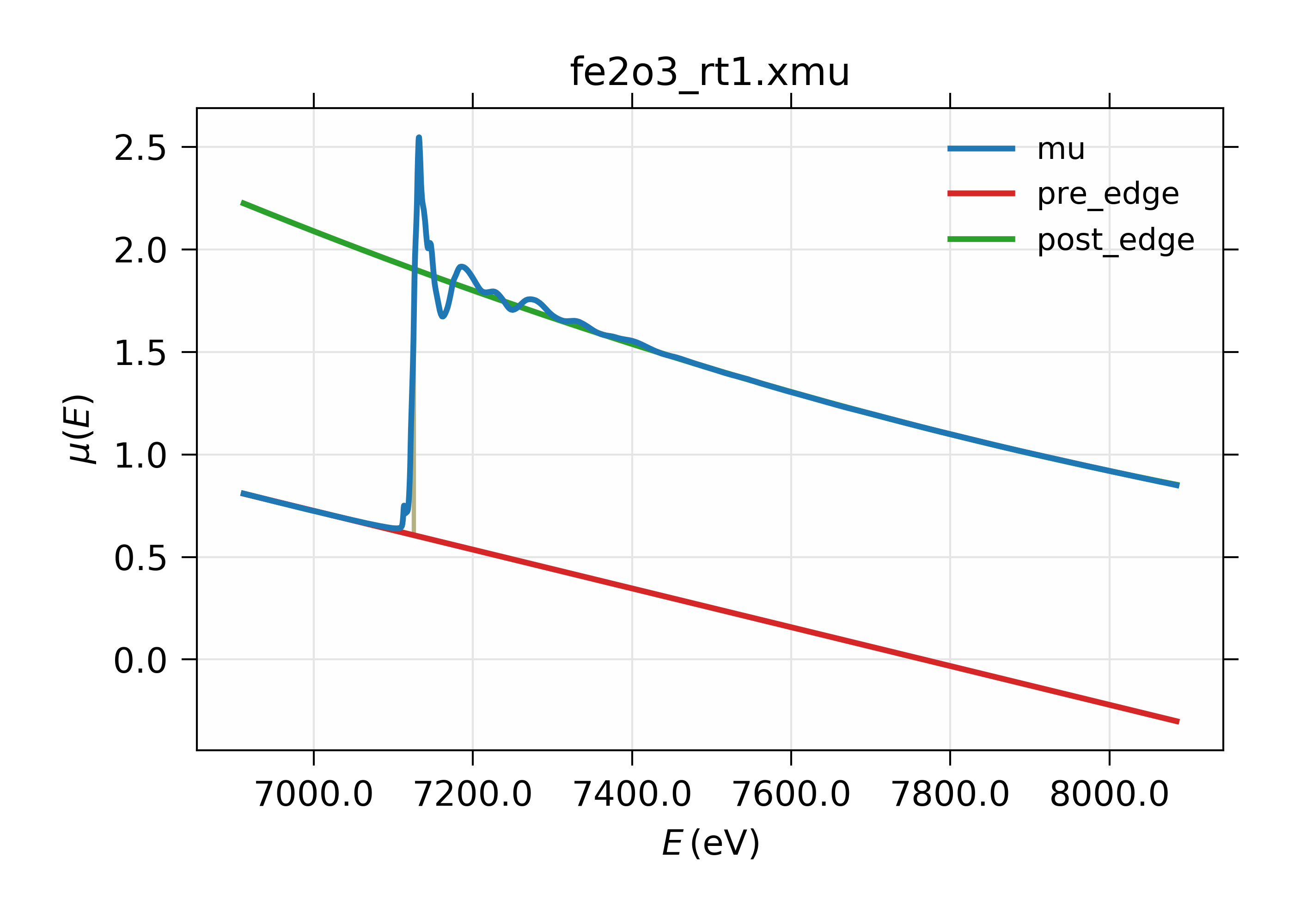Figure 11.2.1 XAFS Pre-edge subtraction.

## 11.2.3. The MBACK algorithm¶

Larch provides an implementation of the MBACK algorithm of Weng [Larch_9] with an option of using the modification proposed by Lee et al [Larch_10]. In MBACK, the data are matched to the tabulated values of the imaginary part of the energy-dependent correction to the Thompson scattering factor, $$f''(E)$$. To account for any instrumental or sample-dependent aspects of the shape of the measured data, $$\mu_{data}(E)$$, a Legendre polynomial of order $$m$$ centered around the absorption edge is subtracted from the data. To account for the sort of highly non-linear pre-edge which often results from Compton scattering in the measurement window of an energy-discriminating detector, a complementary error function is added to the Legendre polynomial.

The form of the normalization function, then, is

$\mu_{back}(E) = \left[\sum_0^m C_i(E-E_0)^i\right] + A\cdot\operatorname{erfc}\left((E-E_{em}\right)/\xi)$

where $$A$$, $$E_{em}$$, and $$\xi$$ are the amplitude, centroid, and width of the complementary error function and $$s$$ is a scaling factor for the measured data. $$E_{em}$$ is typically the centroid of the emission line for the measured edge. This results in a function of $$3+m$$ variables (a tabulated value of $$E_{em}$$ is used). The function to be minimized, then is

$\frac{1}{n_1} \sum_{1}^{n_1} \left[\mu_{tab}(E) + \mu_{back}(E) + s\cdot\mu_{data}(E)\right]^2 + \frac{1}{n_2} \sum_{n_1+1}^{N} \left[\mu_{tab}(E) + \mu_{back}(E) + s\cdot\mu_{data}(E)\right]^2$

To give weight in the fit to the pre-edge region, which typically has fewer measured points than the post-edge region, the weight is adjusted by breaking the minimization function into two regions: the $$n_1$$ data points below the absorption edge and the $$n_2$$ data points above the absorption edge. $$n_1+n_2=N$$, where N is the total number of data points.

If this is used in publication, a citation should be given to Weng [Larch_9].

mback(energy, mu, group=None, ...)

Match measured $$\mu(E)$$ data to tabulated cross-section data.

Parameters: energy – 1-d array of x-ray energies, in eV mu – 1-d array of $$\mu(E)$$ group – output group z – the Z number of the absorber edge – the absorption edge, usually ‘K’ or ‘L3’ e0 – edge energy, $$E_0$$ in eV. If None, the tabulated value is used. emin – the minimum energy to include in the fit. If None, use first energy point emax – the maximum energy to include in the fit. If None, use last energy point whiteline – a margin around the edge to exclude from the fit. If not None, must be a positive integer leexiang – flag for using the use the Lee&Xiang extension [False] tables – ‘CL’ (Cromer-Liberman) or ‘Chantler’, [‘CL’] fit_erfc – if True, fit the amplitude and width of the complementary error function [False] return_f1 – if True, put f1 in the output group [False] pre_edge_kws – dictionary containing keyword arguments to pass to pre_edge(). None.

Follows the First Argument Group convention, using group members named energy and mu. The following data is put into the output group:

attribute meaning
fpp matched $$\mu(E)$$ data
f2 tabulated $$f''(E)$$ data
f1 tabulated $$f'(E)$$ data (if return_f1 is True)
mback_params params group for the MBACK minimization function

Notes:

• The whiteline parameter is used to exclude the region around the white line in the data from the fit. The large spectral weight under the white line can skew the fit result, particularly in data measured over a short data range. The value is eV units.
• The order parameter is the order of the Legendre polynomial. Data measured over a very short data range are likely best processed with order=2. Extended XAS data are often better processed with a value of 3 or 4. The order is enforced to be an integer between 1 and 6.
• A call to pre_edge() is made if e0 is not supplied.
• The option to return $$f'(E)$$ is used by diffkk().

Here is an example of processing XANES data measured over an extended data range. This example is the K edge of copper foil, with the result shown in Figure 11.2.2.

data=read_ascii('../xafsdata/cu_10k.xmu')
mback(data.energy, data.mu, group=a, z=29, edge='K', order=4)
newplot(data.energy, data.f2, xlabel='Energy (eV)', ylabel='matched absorption', label='$f_2$',
legend_loc='lr', show_legend=True)
plot(data.energy, data.fpp, label='Copper foil')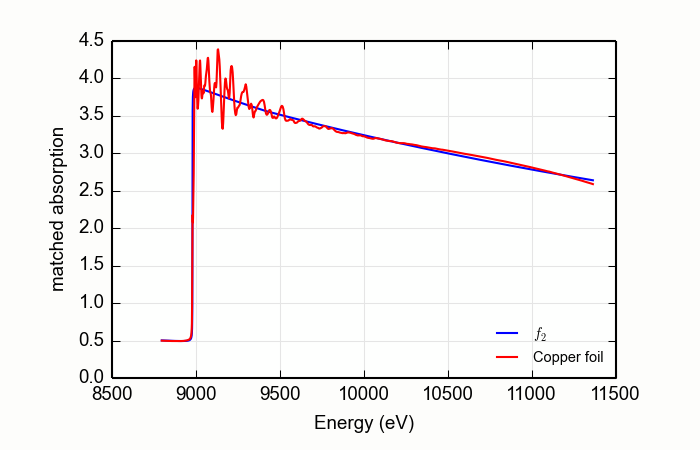Figure 11.2.2 Using MBACK to match Cu K edge data measured on a copper foil.

Here is an example of processing XANES data measured over a rather short data range. This example is the magnesium silicate mineral talc, Mg3Si4O10(OH)2, measured at the Si K edge, with the result shown in Figure 11.2.3. Note that the order of the Legendre polynomial is set to 2 and that the whiteline parameter is set to avoid the large features near the edge.

data=read_ascii('Talc.xmu')
mback(data.e, data.xmu, group=a, z=14, edge='K', order=2, whiteline=50, fit_erfc=True)
newplot(data.e, data.f2, xlabel='Energy (eV)', ylabel='matched absorption', label='$f_2$',
legend_loc='lr', show_legend=True)
plot(data.e, data.fpp, label='Talc ($\mathrm{Mg}_3\mathrm{Si}_4\mathrm{O}_{10}\mathrm{(OH)}_2$)')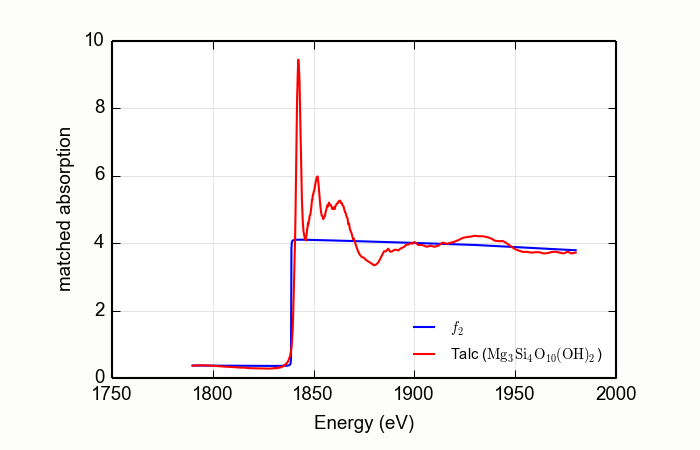Figure 11.2.3 Using MBACK to match Si K edge data measured on talc.

## 11.2.4. Pre-edge baseline subtraction¶

A common application of XAFS is the analysis of “pre-edge peaks” of transition metal oxides to determine oxidation state and molecular configuration. These peaks sit just below the main absorption edge (typically, due to metal 4p electrons) of a main K edge, and are due to overlaps of the metal d-electrons and oxygen p-electrons, and are often described in terms of molecular orbital theory.

To analyze the energies and relative strengths of these pre-edge peaks, it is necessary to try to remove the contribution of the main edge. The main edge (or at least its low energy side) can be modeled reasonably well as a Lorentzian function for these purposes of describing the tail below the pre-edge peaks.

pre_edge_baseline(energy, norm, group=None, form='lorentzian', ...)

remove baseline from main edge over pre edge peak region

This assumes that pre_edge() has been run successfully on the spectra and that the spectra has decent pre-edge subtraction and normalization.

Parameters: energy – array of x-ray energies, in eV, or group (see note 1) norm – array of normalized $$\mu(E)$$ group – output group elo – low energy of pre-edge peak region to not fit baseline [e0-20] ehi – high energy of pre-edge peak region ot not fit baseline [e0-10] emax – max energy (eV) to use for baseline fit [e0-5] emin – min energy (eV) to use for baseline fit [e0-40] form – form used for baseline (see note 2) [‘lorentzian’] with_line – whether to include linear component in baseline [True]

Follows the First Argument Group convention, using group members named energy and norm.

For output, a sub-group name prepeaks is created in the output group (if the output group is None, _sys.xafsGroup will be used), with the following attributes:

attribute meaning
energy energy array for pre-edge peaks = energy[emin:emax]
baseline fitted baseline array over pre-edge peak energies
mu spectrum over pre-edge peak energies
peaks baseline-subtraced spectrum over pre-edge peak energies
dmu estimated uncertainty in peaks from fit
centroid estimated centroid of pre-edge peaks (see note below)
peak_energies list of predicted peak energies (see note belo)
fit_details details of fit to extract pre-edge peaks.

Notes:

• A function will be fit to the input $$\mu(E)$$ data over the range between [emin:elo] and [ehi:emax], ignorng the pre-edge peaks in the region [elo:ehi]. The baseline function is specified with the form keyword argument, which can be one of ‘lorentzian’, ‘gaussian’, or ‘voigt’, with ‘lorentzian’ the default. In addition, the with_line keyword argument can be used to add a line to this baseline function.
• The value calculated for prepeaks.centroid will be found as (prepeaks.energy*prepeaks.peaks).sum() / prepeaks.peaks.sum()
• The values in the peak_energies list will be predicted energies of the peaks in prepeaks.peaks as found by peakutils.

## 11.2.5. Over-absorption Corrections¶

For XAFS data measured in fluorescence, a common problem of over-absorption in which too much of the total X-ray absorption coefficient is from the absorbing element. In such cases, the implicit assumption in a fluorescence XAFS measurement that the fluorescence intensity is proportional to the absorption coefficient of the element of interest breaks down. This is often referred to as self-absorption in the older XAFS literature, but the term should be avoided as it is quite a different effect from self-absorption in X-ray fluorescence analysis. In fact, the effect is more like extinction in that the fluorescence probability approaches a constant, with no XAFS oscillations, as the total absorption coefficient is dominated by the element of interest. Over-absorption most stongly effects the XAFS oscillation amplitude, and so coordination number and mean-square displacement parameters in the EXAFS, and edge-position and pre-edge peak height for XANES. Fortunately, the effect can be corrected for small over-absorption.

For XANES, a common correction method from the FLUO program by D. Haskel ([Larch_11]) can be used. The algorithm is contained in the fluo_corr() function.

fluo_corr(energy, mu, formula, elem, group=None, edge='K', anginp=45, angout=45, **pre_kws)

calculate $$\mu(E)$$ corrected for over-absorption in fluorescence XAFS using the FLUO algorithm (suitabe for XANES, but questionable for EXAFS).

Parameters: energy – 1-d array of x-ray energies, in eV mu – 1-d array of $$\mu(E)$$ formula – string for sample stoichiometry group – output group elem – atomic symbol (‘Zn’) or Z of absorbing element edge – name of edge (‘K’, ‘L3’, …) [default ‘K’] anginp – input angle in degrees [default 45] angout – output angle in degrees [default 45] pre_kws – additional keywords for pre_edge(). None

Follows the First Argument Group convention, using group members named energy and mu. The value of mu_corr and norm_corr will be written to the output group, containing $$\mu(E)$$ and normalized $$\mu(E)$$ corrected for over-absorption.

## 11.2.6. Spectral deconvolution¶

In order to readily compare XAFS data from different sources, it is sometimes necessary to considert the energy resolution used to collect each spectum. To be clear, the resolution of an EXAFS spectrum includes contributions from the x-ray sourse, instrumental broadening from the x-ray optics (especially the X-ray monochromator used in most measurements), and the intrinsic lifetime of the excited core electronic level. For data measured in X-ray fluorescence or electron emission mode, the energy resolution can also includes the energy width of the decay channels measured.

For a large fraction of XAFS data, the energy resolution is dominated by the intrinsic width of the excited core level and by the resolution of a silicon (111) double crystal monochromator, and so does not vary appreciably between spectra taken at different facilities or at different times. Exceptions to this rule occur when using a higher order reflection of a silicon monochromator or a different monochromator altogether. Resolution can also be noticeably worse for data taken at older (first and second generation) sources and beamlines, either without a collimating mirror or slits before the monochromator to improve the resolution. In addition, high-resolution X-ray fluorescence measurements can be used to dramatically enhance the energy resolution of XAFS spectra, and are becoming widely available.

Because of these effects, it is sometimes useful to change the resolution of XAFS spectra. For example, one may need to reduce the resolution to match data measured with degraded resolution. This can be done with xas_convolve() which convolves an XAFS spectrum with either a Gaussian or Lorentzian function with a known energy width. Note that convolving with a Gaussian is less dramatic than using a Lorenztian, and usually better reflects the effect of an incident X-ray beam with degraded resolution due to source or monochromator.

One may also want to try to improve the energy resolution of an XAFS spectrum, either to compare it to data taken with higher resolution or to better identify and enumerate peaks in a XANES spectrum. This can be done with xas_deconvolve() function which deconvolves either a Gaussian or Lorentzian function from an XAFS spectrum. This usually requires fairly good data. Whereas a Gaussian most closely reflects broadening from the X-ray source, broadening due to the natural energy width of the core levels is better described by a Lorenztian. Therefore, to try to reduce the influence of the core level in order better mimic high-resolution fluorescence data, deconvolving with a Lorenztian is often better.

### 11.2.6.1. xas_convolve()¶

xas_convolve(energy, norm=None, group=None, form='lorentzian', esigma=1.0, eshift=0.0):

convolve a normalized mu(E) spectra with a Lorentzian or Gaussian peak shape, degrading separation of XANES features.

This is provided as a complement to xas_deconvolve, and to deliberately broaden spectra to compare with spectra measured at lower resolution.

Parameters: energy – 1-d array of $$E$$ norm – 1-d array of normalized $$\mu(E)$$ group – output group form – form of deconvolution function. One of ‘lorentzian’ or ‘gaussian’ [‘lorentzian’] esigma – energy $$\sigma$$ (in eV) to pass to gaussian() or lorentzian() lineshape [1.0] eshift – energy shift (in eV) to apply to result. [0.0]

Follows the First Argument Group convention, using group members named energy and norm. The following data is put into the output group:

attribute meaning
conv array of convolved, normalized $$\mu(E)$$

### 11.2.6.2. xas_deconvolve()¶

xas_deconvolve(energy, norm=None, group=None, form='lorentzian', esigma=1.0, eshift=0.0, smooth=True, sgwindow=None, sgorder=3)

XAS spectral deconvolution

de-convolve a normalized mu(E) spectra with a peak shape, enhancing the intensity and separation of peaks of a XANES spectrum.

The results can be unstable, and noisy, and should be used with caution!

Parameters: energy – 1-d array of $$E$$ norm – 1-d array of normalized $$\mu(E)$$ group – output group form – form of deconvolution function. One of ‘lorentzian’ or ‘gaussian’ [‘lorentzian’] esigma – energy $$\sigma$$ (in eV) to pass to gaussian() or lorentzian() lineshape [1.0] eshift – energy shift (in eV) to apply to result. [0.0] smooth – whether to smooth the result with the Savitzky-Golay method [True] sgwindow – window size for Savitzky-Golay function [found from data step and esigma] sgorder – order for the Savitzky-Golay function 

Follows the First Argument Group convention, using group members named energy and norm.

Smoothing with savitzky_golay() requires a window and order. By default, window = int(esigma / estep) where estep is step size for the gridded data, approximately the finest energy step in the data.

The following data is put into the output group:

attribute meaning
deconv array of deconvolved, normalized $$\mu(E)$$

### 11.2.6.3. Examples using xas_deconvolve() and xas_convolve()¶

An example using xas_deconvolve() to deconvolve a XAFS spectrum would be:

## examples/xafs/doc_deconv1.lar
dat = read_ascii('../xafsdata/fe2o3_rt1.xmu', labels='energy mu i0')

pre_edge(dat)
xas_deconvolve(dat, esigma=1.0)

plot_mu(dat, show_norm=True, emin=-30, emax=70)
plot(dat.energy, dat.deconv, label='deconvolved')

## end of examples/xafs/doc_deconv1.lar


resulting in deconvolved data:Figure 11.2.4 Deconvolved XAFS spectrum for $$\rm Fe_2O_3$$.

To de-convolve an XAFS spectrum using the energy width of the core level, we can use the _xray.core_width() functiion, as shown below for Cu metal. We can also test that the deconvolution is correct by using xas_convolve() to re-convolve the result and comparing it to original data. This can be done with:

## examples/xafs/doc_deconv2.lar

dat = read_ascii('../xafsdata/cu_metal_rt.xdi', labels='energy i0 i1 mu')
dat.mu = -log(dat.i1 / dat.i0)

pre_edge(dat)

esigma = core_width('Cu', edge='K')

xas_deconvolve(dat, esigma=esigma)

plot_mu(dat, show_norm=True, emin=-50, emax=250)
plot(dat.energy, dat.deconv, label='deconvolved')

# Test convolution:
test = group(energy=dat.energy, norm=dat.deconv)
xas_convolve(test, esigma=esigma)
plot_mu(dat, show_norm=True, emin=-50, emax=250, win=2)
plot(test.energy, 100*(test.conv-dat.norm),
label='(reconvolved - original)x100', win=2)
## end of examples/xafs/doc_deconv2.lar


with results shown below:Figure 11.2.5 Cu metal normalized $$\mu(E)$$ and spectrum deconvolved by the energy of its core level.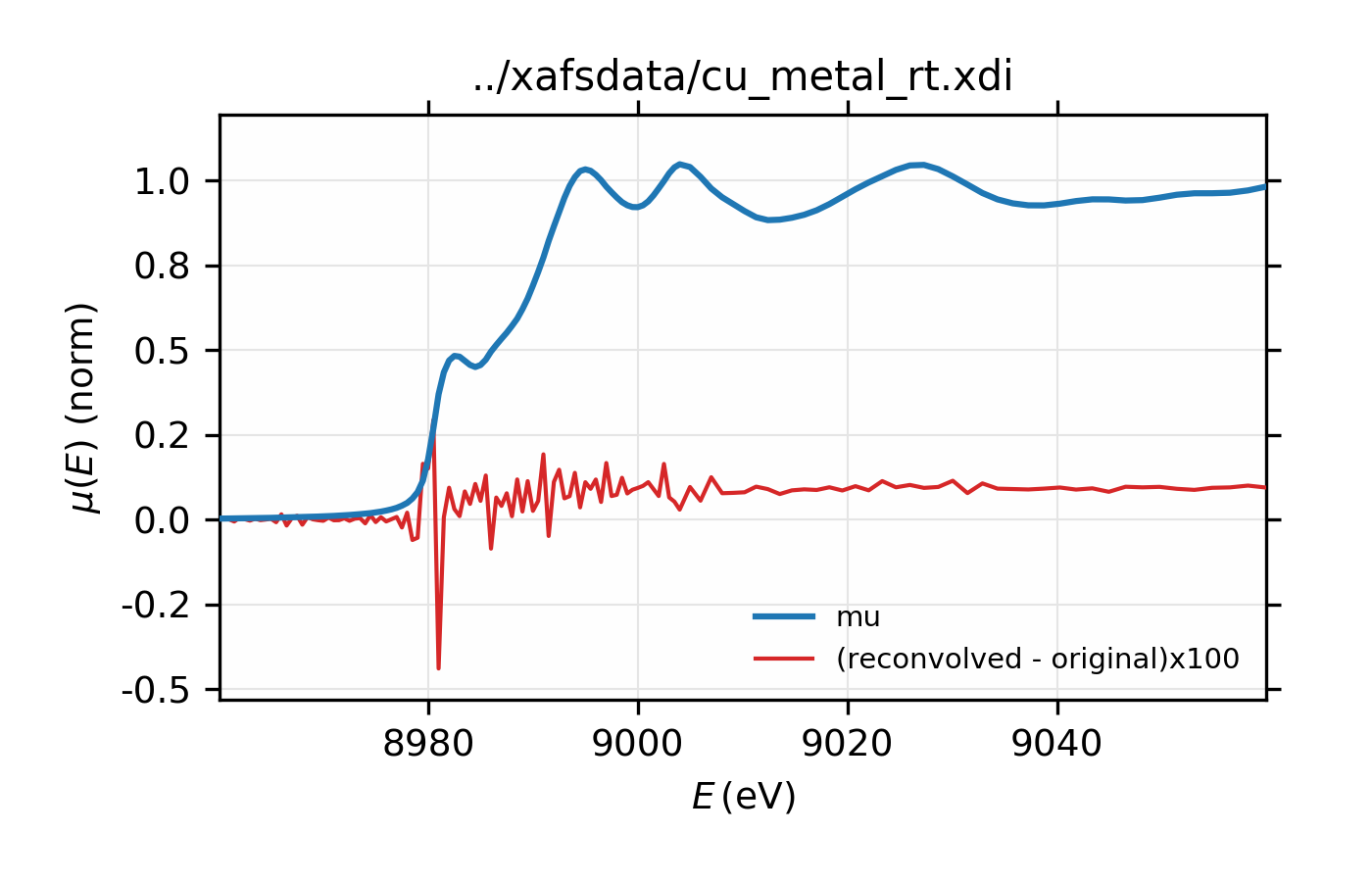Figure 11.2.6 Comparison of original and re-convolved spectrum for Cu metal. The difference shown in red is multiplied by 100.

Example of simple usage of xas_deconvolve() and xas_convolve() for Cu metal.

Finally, de-convolution of $$L_{\rm III}$$ XAFS data can be particularly dramatic and useful. As with the copper spectrum above, we’ll deconvolve $$L_{\rm III}$$ XAFS for platinum, using the nominal energy width of the core level (5.17 eV). For this example, we also see noticeable improvement in amplitude of the XAFS.

## examples/xafs/doc_deconv3.lar

data = read_ascii('../xafsdata/pt_metal_rt.xdi', labels='energy time i1 i0')
data.mu = -log(data.i1 / data.i0)

pre_edge(data)
autobk(data, rbkg=1.1, kweight=2)
xftf(data, kmin=2, kmax=17, dk=5, kwindow='kaiser', kweight=2)

xas_deconvolve(data, esigma=core_width('Pt', edge='L3'))

decon = group(energy=data.energy, mu=data.deconv, filename=data.filename)
autobk(decon, rbkg=1.1, kweight=2)
xftf(decon, kmin=2, kmax=17, dk=5, kwindow='kaiser', kweight=2)

# plot in E
plot_mu(data, show_norm=True, emin=-50, emax=250)
plot(data.energy, data.deconv, label='deconvolved',  win=1)

# plot in k
plot_chik(data, kweight=2, show_window=False, win=2)
plot_chik(decon, kweight=2, show_window=False,
label='deconvolved', new=False, win=2)

# plot in R
plot_chir(data,  win=3)
plot_chir(decon, label='deconvolved', new=False, win=3)
## end examples/xafs/doc_deconv3.lar


with results shown below: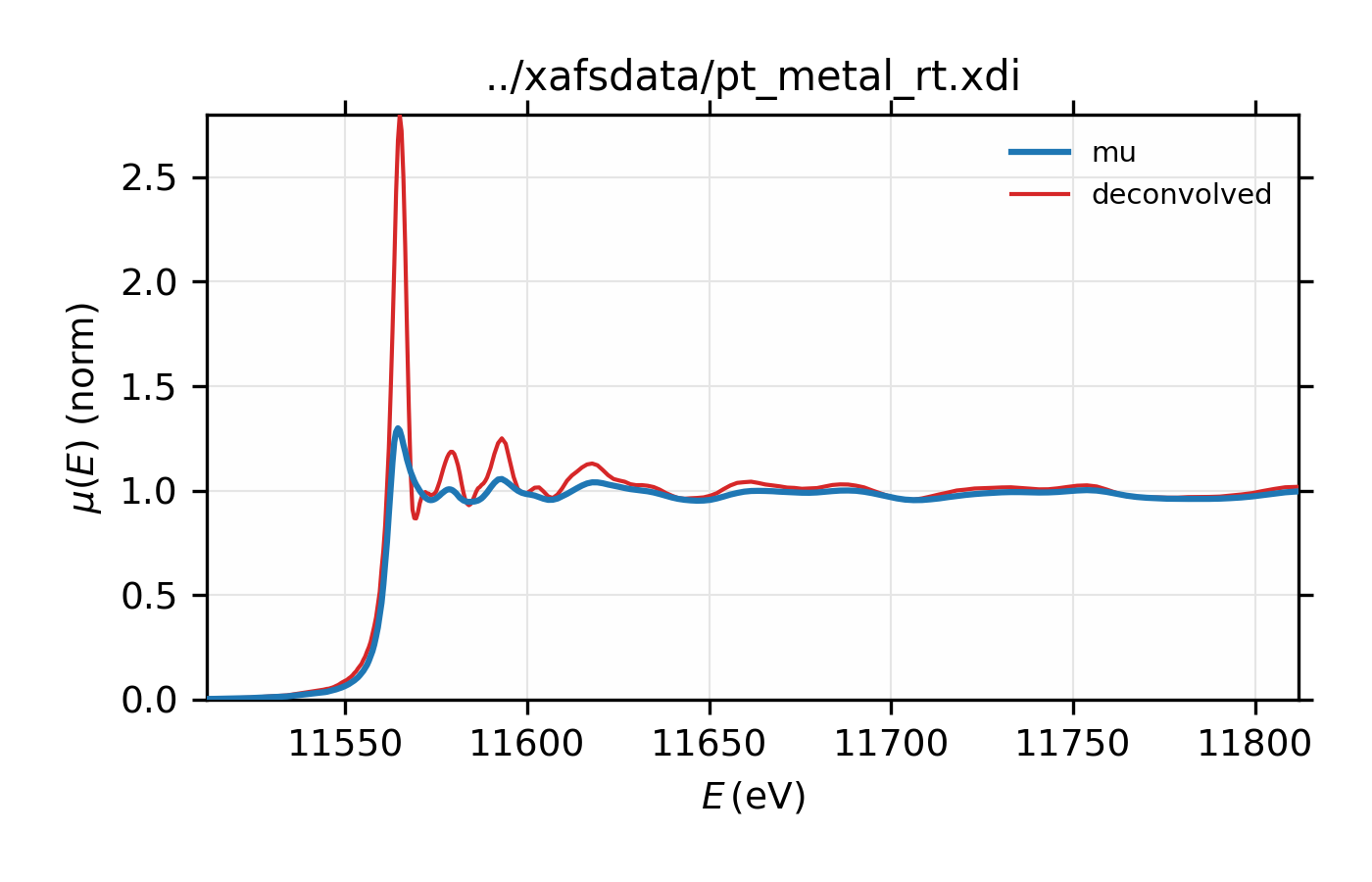Figure 11.2.7 $$L_{\rm III}$$ XAFS of Pt metal, normalized $$\mu(E)$$ for raw data and the spectrum deconvolved by the energy of its core level.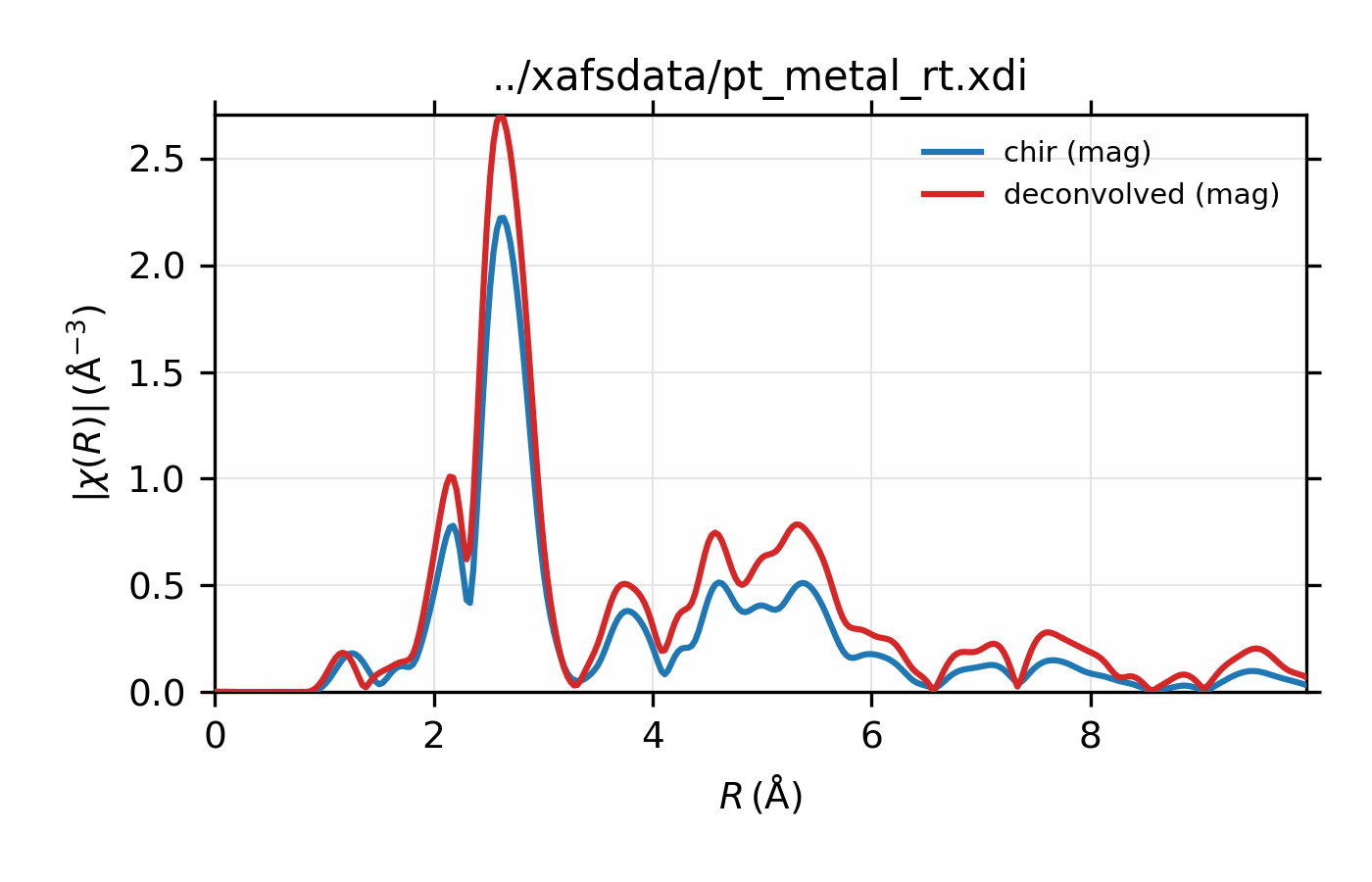Figure 11.2.8 $$L_{\rm III}$$ XAFS of Pt metal, $$\chi(R)$$ for raw data and the spectrum deconvolved by the energy of its core level.

Example of simple usage of xas_deconvolve() and xas_convolve() for $$L_{\rm III}$$ XAFS of Pt metal.

References

 [Larch_9] (1, 2) Tsu-Chien Weng, Geoffrey S. Waldo, and James E. Penner-Hahn. A method for normalization of X-ray absorption spectra. Journal of Synchrotron Radiation, 12(4):506–510, Jul 2005. doi:10.1107/S0909049504034193.
 [Larch_10] Julia C. Lee, Jingen Xiang, Bruce Ravel, Jeffrey Kortright, and Kathryn Flanagan. Condensed matter astrophysics: a prescription for determining the species-specific composition and quantity of interstellar dust using x-rays. The Astrophysical Journal, 702(2):970, 2009. URL: http://stacks.iop.org/0004-637X/702/i=2/a=970, doi:0004-637X/702/i=2/a=970.
 [Larch_11] D. Haskel. Fluo. http://www.aps.anl.gov/~haskel/fluo.html, 1999.